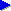Home# Grouping bytes to make common data types and sizes

On the previous page, we introduced the 8-bit byte as the fundamental unit of data storage. A byte can hold one of 256 different values (256 because the byte has 8 bits each with 2 possible values, giving 2*2*2*2*2*2*2*2 or 28 =256 possibilities). Historically, this has emerged as a convenient size for common data elements such as a character or a colour component of an image pixel. But of course, 256 possible values isn't enough for various types of data. In such circumstances, a number of bytes are generally combined to form larger data types.

## 2-byte values (short, half word)

If we combine 2 bytes, we get a value with 16 bits which can store up to 65536 distinct values (=256*256). In Java, the short data type holds 16 bits. In some other languages, notably C, it's common to refer to a 16-bit data type as a short or short integer.

The term word is sometimes used to refer to a group of several bytes that form a unit or number. On some systems, there is a convention that a "word" consists specifically of four bytes, so that a two-byte grouping is called a half word. This term isn't universal, though.

16-bit values are typically used in cases where the value we need to store can have a "few thousand" values, such as:

• the X or Y screen co-ordinate of a pixel;
• a sound sample on a CD or DVD;
• a typical character of Unicode, a common character encoding system that allows for a wide variety of characters (e.g. Chinese and Japanese characters, phonetic symbols etc) to be encoded.

## 4-byte values (word, int)

If we combine 4 bytes, we get a value with 32 bits, which can store 65536*65536 distinct values. This gives a range of approximately 4 billion different values. In many languages, a data type referred to simply as an "int" (=integer) is assumed to be 4 bytes. In Java, the data type int is defined precisely to be four bytes.

As just mentioned, on some systems, there is a convention that a word is precisely four bytes (but this isn't universal).

On many modern processors, a 4-byte value is the size of value that the CPU most "conveniently" processes (that is, it is the register size, or size of the "internal variables" of the processor).

4-byte integers are generally used in cases such as:

• to perform whole-number arithmetic for most "normal" purposes, where there's no specific need for a large number (where by "large", we mean values bigger than a couple of billion);
• as the normal size of number to store where we've no specific need for another size;
• to refer to a memory address on most systems;
• in cases where it's convenient to treat a group of four bytes together: for example, a 4-byte word can hold a pixel colour value consisting of one byte each for the read, green, blue and transparency components; or we could combine the left and right samples of a stereo 16-bit sound source into one 4-byte word per stereo sample.

## 8-byte values (long, double word)

Occasionally, a 4-byte integer with its range of 4 billion or so distinct values is not enough. In such cases, it's common to combine a total of 8 bytes. Since it's double the capacity of a 4-byte integer, this gives a total of around 16 billion billion distinct values. In Java, an 8-byte number is called a long. Longs are mainly used in the following cases:

• for file sizes or file offsets: using a 4-byte word for a file size (which was once common), allows a maximum file size of "only" about 4 billion bytes, or about 4 gigabytes. In some applications, such as databases, having files larger than this is not uncommon;
• for timestamps: it's common to take timings as the number of milliseconds (thousandths of a second) since a given point; since there are over 86 million milliseconds in a day, restricting a timestamp to 4 bytes would not allow us to time a period of very many days or store times within a very large range.

The size of 8 bytes is also one that many processors can "conveniently" handle in some way. For some (so-called 64-bit processors), it is the size that processor 'naturally' handles. And even in the case of processors that most naturally handle 4 byte values (32-bit processors), these usually have some operations that deal with 64-bit values, for example the ability to multiply two 32-bit values together and give the result as a 64-bit value.

## Other group sizes

It's possible to store a number as any aribitrary number of bits or bytes that is convenient. For example, if we needed a number large than 4 bytes, but 8 bytes was overkill, we might opt for 6 bytes.

Nowadays, it's becoming less and less common to stray beyond the "power of 2" (1*2 = 2 bytes, 2*2 = 4 bytes, 4*2 = 8 bytes) sizes mentioned. Usually, especially in the case of 4 or 8 bytes, modern processors and programming languages are designed to work efficiently with these sizes of number. So with the lagre memory and storage capacities of modern computers, it's usually not worth the extra programming effort to use a non-standard size just to shave a few bytes off here and there.